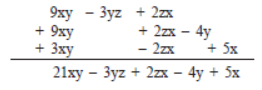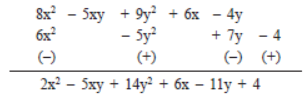Courses

# Points to Remember - Algebraic Expressions and Identities Class 8 Notes | EduRev

## Class 8 Mathematics by Full Circle

Created by: Full Circle

## Class 8 : Points to Remember - Algebraic Expressions and Identities Class 8 Notes | EduRev

The document Points to Remember - Algebraic Expressions and Identities Class 8 Notes | EduRev is a part of the Class 8 Course Class 8 Mathematics by Full Circle.
All you need of Class 8 at this link: Class 8

Points to Remember

• Expressions containing one, two or three terms are called monomial, binomial and trinomial respectively.
• Any expression having one or more terms is called a polynomial.
• Any monomial multiplied by a monomial gives a monomial.
• The numerical factor of a term is called its coefficient.
• An identity is an equality, which is true for all values of the variables in the equality.
• The standard identities are:
(a + b)2 = a2 + 2ab + b2                  …(i)
(a – b)2 = a2 – 2ab + b2                  …(ii)
(a + b)(a – b) = a2 – b2                   …(iii)
(x + a)(x + b) = x2 + (a + b)x + ab   …(iv)

We Know That
Algebraic expressions are formed from the variables and constants. A variable can take any value. The value of an expression changes with the value chosen for the variables it contains.
A number line has countless points. A variable can take position on the number line.

Like and Unlike Terms
The terms having the same literal factors are called like (or similar) terms, otherwise they are called unlike (or dissimilar) terms.

Addition and Subtraction of Algebraic Expressions
Example 1. Add: 9xy – 3yz + 2zx;  2xz + 9xy – 4y and –2xz + 5x + 3xy.
Solution: We write the expressions in separate rows, keeping like terms in the same column:Note:
I. In the above example, –4y and 5x are carried over as they are, because they have no like terms.
II. xz is same as zx.

Example 2. Subtract 6x2 – 5y2 + 7y – 4 from 8x2 – 5xy + 9y2 + 6x – 4y
Solution: We know that subtraction of a number is the same as addition of its additive inverse.
We haveExample 3. Which of the following is a binomial?
a. 8*a+a
b. 7a2 + 8b + 9c
c. 3a*4b + 2c
d. 11a2 + 11b
Solution:
d. 11a2 + 11b
a) will give 8a+a=9a which is monomial.

b) is a trinomial

c) will give 24abc, which is a monomial

d) will give 11a2+11b2, which is a binomial

Example 4. Identify the variable, exponent, and coefficient in the given term of 3/5 k8
Solution:
Here the variable is k, the exponent is 8 and coefficient is 3/5.

Example 5. Identify the variable and constant in the expression 24 – x.
Solution:
In the expression above, 24 is the constant and x is the variable since 24 is fixed and cannot change its value whereas x can be anything.

93 docs|16 tests

,

,

,

,

,

,

,

,

,

,

,

,

,

,

,

,

,

,

,

,

,

;